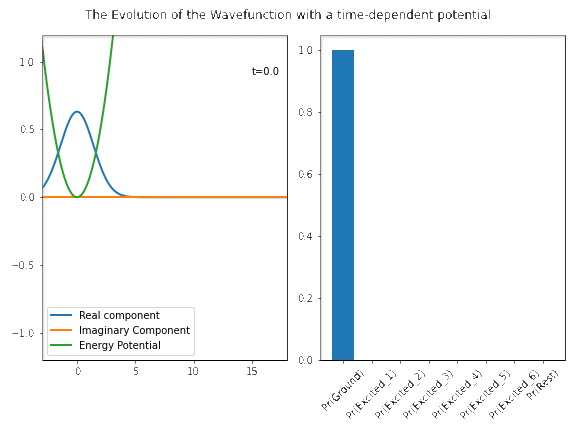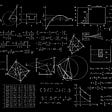# Quantum Data and its Embeddings #1

Understand Data Embedding concept in Quantum Computing

This article describes Data embedding fundamentals in simple way, so that, it may be useful for Quantum Algorithms and Quantum Machine Learning Algorithms. Users should be familiar with Quantum computing concepts.

As we know 8085 is an 8-bit microprocessor and stores the data in the form of 8-bits, in the similar way we embedd the data into quantum bits (Qubits) for Quantum Computing, so that new computation model (QC) execute our programs efficiently.

## Classical computing data

Bit is unit of information stored and processed in Classical Computing (Binary Digital system).

Classical computers utilize the binary or 2-bit system. 2-bits are 1 and 0. 2-bit system is considered to be a computer processing or computing the data and instructions or programs from the user and uses 0 and 1 for execution. Classical computers are the one that we use today. Todays classical computers are smartphones, laptops, desktop and servers.

## Quantum computing data

Quantum computers introduce 3-bit or base-3 system. Quantum computers are made up of the laws of Quantum physics. Quantum computers uses binary bits (0 and 1) along with the option of the bit being both 0 and 1 at the same time (superposition), this creates the third state. Eventually 3 states are 0,1 and 0–1.

Quantum bit or Qubit is the basic unit of quantum information, that can be in a state of both 0 and 1 at the same time. The 3rd state creates more processing power. How states are processing in 3-bit systems described below.

## State processing in quantum computers

As you know in quantum computing if there are ’n’ qubits you will have

basis states. In this way, Quantum Computing process all basis states in a single time. For example, if 2 qubits present its basis states are 00,01,10 and 11 and processed all these states using its amplitudes or probabilities is as follows.

## Data representation in Classical and Quantum systems

Below figure describes how classical data embedding into single Qubit.

As Bloch sphere is considered to be represent the data in a Qubit. The Bloch sphere is a geometrical representation of pure single-qubit states as a point on the unit sphere.

Well, Quantum Computers has not to be applied for classical data, in order to gain its power we have to provide the Quantized data. There are many patterns or encoding or embedding techniques exists to load classical data into Quantum computers.

## Encoding

Working directly with classical data is not possible and inefficient as well in Quantum Computing. Quantum Computers has to be compatible with data and data has to be in the form of Quantum Laws like Superposition and Entanglement. Some Quantum experiments produce the data which follows quantum laws with bit noisy, we have to use embedding techniques to cleanse the data and give it in the expected format for quantum algorithms to process further.

Loading data into a quantum computer is not trivial. To load the data, it must be encoded in quantum bits (Qubits). Mapping classical data points into n-qubit Quantum States.

The below figure shows Encoding overview and how it appears in designing circuit.

## Processing

Processing is the step where Quantum embedded input processed. For any Quantum Algorithm (or Quantum AI algorithm) it is in the form of quantum circuit, it can be variational circuit or a quantum routine.

## Measurement or Prediction

This is the step where the expected outcome will come. Ultimately, measuring the qubit and obtain the result in the form of scalar. This step becomes the prediction for any Quantum Algorithm.

Data Loading is a function which maps classical data point into n-qubit quantum states.

For example, if there are 8 basis states and transferring it into 3 qubit quantum state. This loading process can be processed by a Quantum Circuit (in Machine learning perspective it is Quantum Neural Networks (QNN)).

Quantum computers naturally map data into Hilbert space. The map that performs the embedding has been termed a quantum feature map.

## What does Quantum embedding do?

A Quantum embedding is then the representation of classical data points ‘x’ as quantum states facilitated by the feature map.

## Quantum Feature Map

Encoding data into quantum states is a feature map. Feature map transform the data into a new space where it is linear by way of feature map. In Linear algebra, new space is called Hilbert Space.

More formally, it is defined as a function which maps input data into feature space.

The outputs of the map on the individual data points are called feature vectors. i.e.,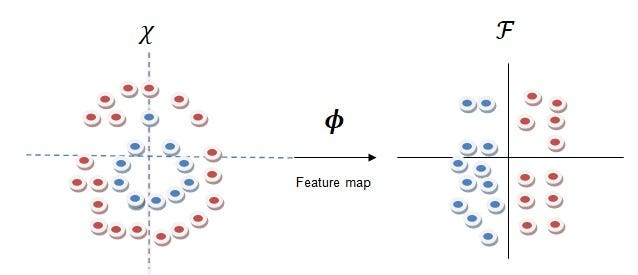Feature map can transform data into Hilbert space where it can be easily to separable

In the output i.e., Hilbert space is a vector space and feature vectors are “Quantum states”. This mapping can transforms by the way of a unitary transformation, i.e.,

Encoding mechanism involves Decomposition methodologies in Linear Algebra. It involves a bit Matrix Decompositions, Unitary transformations, Hilbert spaces, inner products, etc.,

## How the data encoded in Hilbert spaces?

As we know that Feature space is Hilbert space, and need to see how the data is encoding in Qubits.

Training the embedding and finally store the data in Hilbert space i.e., Quantum Hilbert Space. In the below examples figures explores different kinds of classical data embedd into quantum Hilbert spaces.

## Example 1

In this research paper, how data embedded in Hilbert spaces along with various definitions, kernel methods in ML Algorithms, etc., very well.

## Example 2

In this example, data embedding into Hilbert spaces, and can be viewed geometrical sphere, which differentiates two classes of data into 2 Hilbert spaces and ultimately stores embed data into 2-qubit system contains 4 basis states.

Example 3

In the below example (first part describes) how classical data is embedded into Hilbert space for various data points and converted into unique Hilbert space accordingly. (kindly note that feature vector should be in the form of quantum state vector).There are 3 different types of Data points and converted into 3 different Hilbert spaces. So, embed data stores into 3-qubit systems and contain 8-basis states in quantum state (i.e., quantum state vectors whose length is 8).

Second part deals with circuit design i.e., Quantum Circuit for embedd (preprocessing) and classification of the new data points into 3 categories.

From the first example Data embedded into vector space as two different Hilbert spaces. As we know the Hilbert space definition “A Complete space with an inner product.” and written in Dirac notation as

Because of the probabilistic interpretation influenced in quantum mechanics, state vectors (feature vectors or quantum state vectors) are normalized to 1.

## Machine Learning ‘s Binary Classification Example in Quantum Neural Network. — Circuit Design for embedd and classification.

Consider the data points and associated classes ‘A’ and ‘B’ of labels 1 and 2, respectively, and have to predict the label of a new input ‘x’ which is not in the training set.

Below figure shows how the the data is embedd in hidden layer and then forward to classify the encoded data in the output layer, all these combined into Neural Network i.e., Quantum Neural Network (QNN).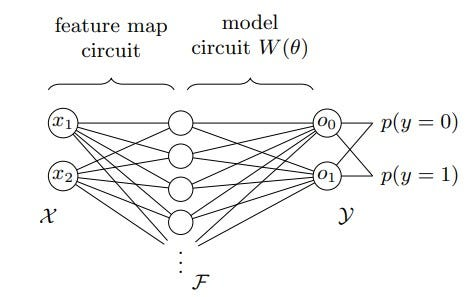QNN shows data embedding in Hilbert Feature Space and Binary class

## Space and Time complexity of Data Encoding

Let us discuss the notations of Classical and Quantum data in order to understand space and time complexity for encoding. Generally, Big ‘O’ notation is preferred for estimating the complexity of space and time. Big ‘O’ notation allows us to estimate the algorithm performance in best and worst cases.

The beauty of Big ‘O’ notation is that it can be used in various fields for various purposes, but, in Quantum Computing, Big ‘O’ is used for determining the required number of qubits for embedding. Ultimately, our classical data gathered from various sources and it has to be encode into quantum data, and quantum data has to store and process in the form of Qubits.

Classical Data has ‘M; data points, features are x’s and labels y’s. Each input has ’N’ features and our task is to map each data point to n-qubit quantum state.

Qubits defined as ‘n’, qubits can represent a data point with ‘N’ features. This formula provides an exponential saving in “space” at the cost of exponential increase in “time”. In general, this quantum state takes time .

Data encoding done with logarithmic base 2. For example,

That is, a quantum state of n qubits represents a data point with N features.

In general, such a quantum state takes qubits (Big O 2 power n) formula to prepare. i.e.,

In practice, classical data is encoded via a state preparation circuit. In Machine learning perspective, circuit is Quantum Neural Network.

There are various techniques used in Data Loading and they are Basis encoding, Amplitude encoding, Angle encoding, QuAM (Quantum Associative Memory), QSample encoding, Divide-and-conquer encoding, QRAM and (Quantum Random Access Memory) and more along with variants. It is allowed to apply multiple encodings.

The loading is accomplished by an encoding from set X (classical data contains data points) to n-qubit quantum states.

## Why do we move to Data Embedding patterns?

As such, this much of time complexity makes algorithms to be worse, to overcome this type of difficulty there are various Data Embedding techniques involved and we need to select particular pattern which suits our Algorithms. For instance, various Data encodings and their run time described pictorially.

Data embedding techniques or patterns come close to execution time lesser than above described.

Data embedding techniques along with space and time complexity will be discussed in the #2 article.

## Conclusion

Hope the reader get clear picture about state processing, data encoding in qubits, feature map, data in Hilbert spaces, and why we need to move other data encoding techniques.

Thanks for reading my article. Give a clap if it helps to you, let me know if there are any mistakes.

--

--

--

## More from MLearning.ai

Data Scientists must think like an artist when finding a solution when creating a piece of code. ⚪️ Artists enjoy working on interesting problems, even if there is no obvious answer ⚪️ linktr.ee/mlearning 🔵 Follow to join our 18K+ Unique DAILY Readers 🟠

## Recommended from Medium## Essential Guide to Auto-Encoders in Data Science (Part 1)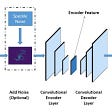## Listings similarities using leaves## Sentiment analysis in less than 50 lines of Python## Using Tensorflow 2.0 to Build a CNN for Image Classification 😎## Triple Crossover: Alibaba’s CrossE Improves Knowledge Graph Embedding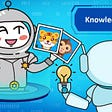## Personalization @Intuit Part 3 — Platform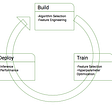## Some Things Uber Learned from Running Machine Learning at Scale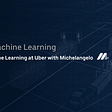## Shafi

Data Architect, Researcher in AI & Quantum Computing. Passionate in Autonomous.

## Comparing Statistical Models for Randomized Benchmarking in Qiskit